## 关于

1. 什么是过拟合与泛化？为什么要划分训练集、测试集、验证集。
2. 什么是交叉验证？
3. 如何评估一个分类模型的好坏？
4. 方差-偏差分解
5. 决策树的减枝与泛化

1. 至少有高中数学水平。
2. 了解决策树，如果还不了解，可以参看决策树模型
3. 如果你需要完成实践部分，需要有基本的 python 知识，你可以通过python快速入门快速了解python如何使用。

sepal length (cm) sepal width (cm) petal length (cm) petal width (cm) target
91 6.1 3.0 4.6 1.4 1
77 6.7 3.0 5.0 1.7 1
99 5.7 2.8 4.1 1.3 1
65 6.7 3.1 4.4 1.4 1
14 5.8 4.0 1.2 0.2 0
108 6.7 2.5 5.8 1.8 2
142 5.8 2.7 5.1 1.9 2
127 6.1 3.0 4.9 1.8 2
24 4.8 3.4 1.9 0.2 0
2 4.7 3.2 1.3 0.2 0

• sepal length (cm) 萼片长度
• sepal width (cm) 萼片宽度
• petal length (cm) 花瓣长度
• petal width (cm) 花瓣宽度

• 0： setosa 山鸢尾
• 1： versicolor 变色鸢尾
• 2： virginica 维吉尼亚鸢尾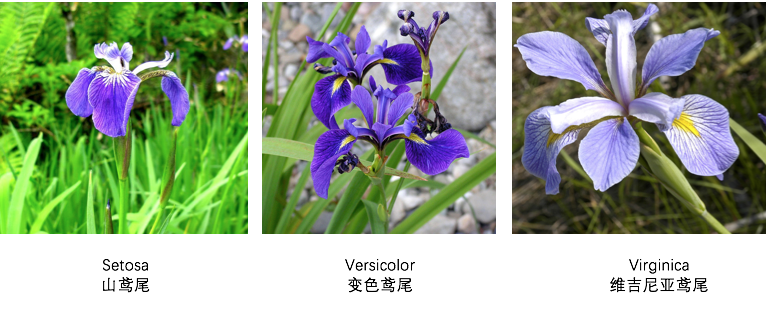## 分类准确率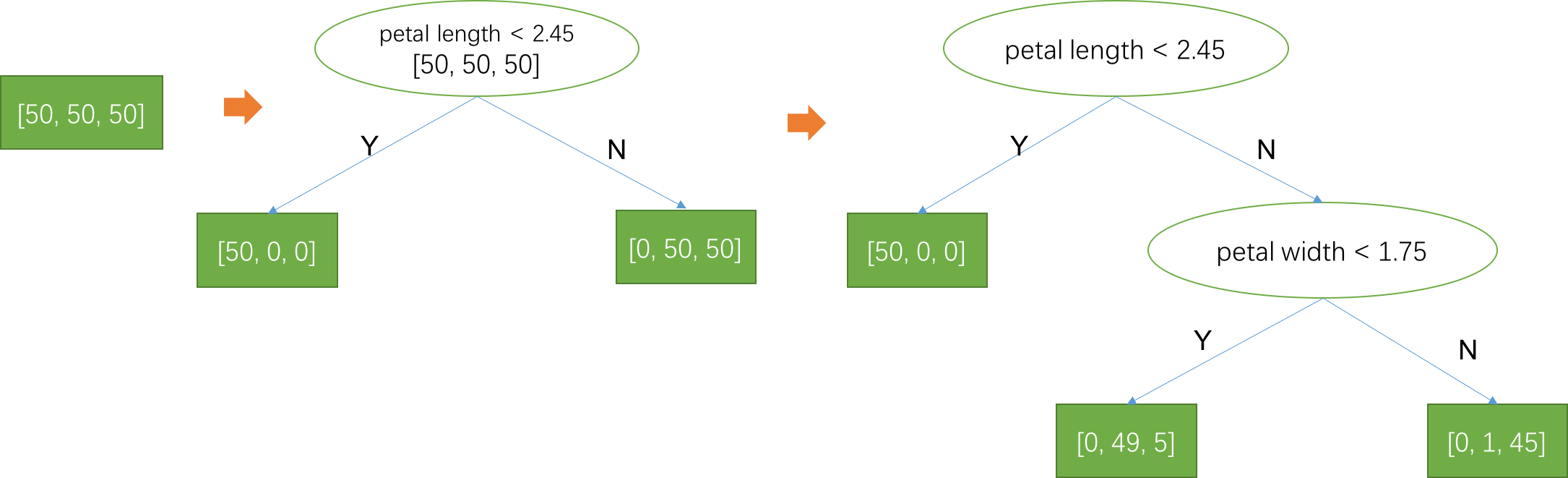## 交叉验证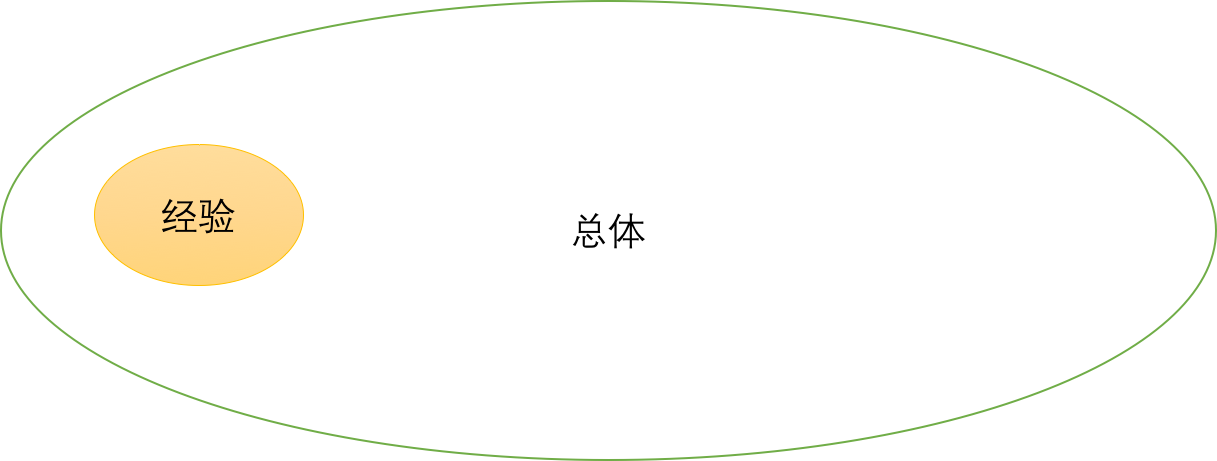$$\hat{P} _ c = E_{(x, y) \sim D} I(h(x) = y)$$

$$P _ c = E_{(x, y) \sim P(x, y)} I(h(x) = y)$$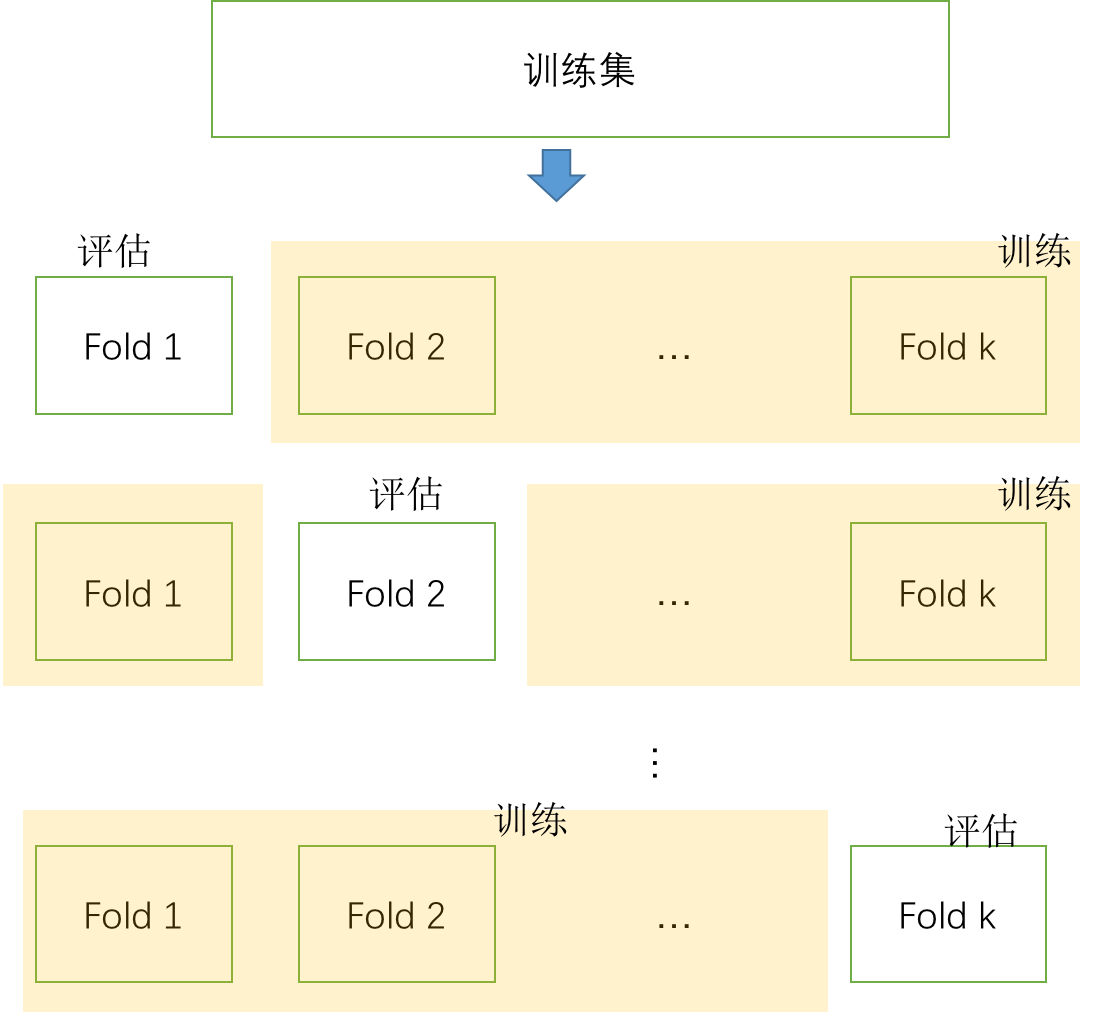## 过拟合与决策树减枝### 过拟合的方差偏差解释

\begin{align} E[(y - \hat{f}(x))^2] &= E[y^2 + \hat{f}^2 - 2y\hat{f}] \\ &=Var[y]+ Var[\hat{f}] +[Ey]^2 +[E\hat{f}]^2 - 2 f E\hat{f} \\ &= Var[y] + Var[\hat{f}] + (f - E\hat{f})^2 \\ &= \sigma^2 + Var[\hat{f}] + Bias[\hat{f}]^2 \end{align}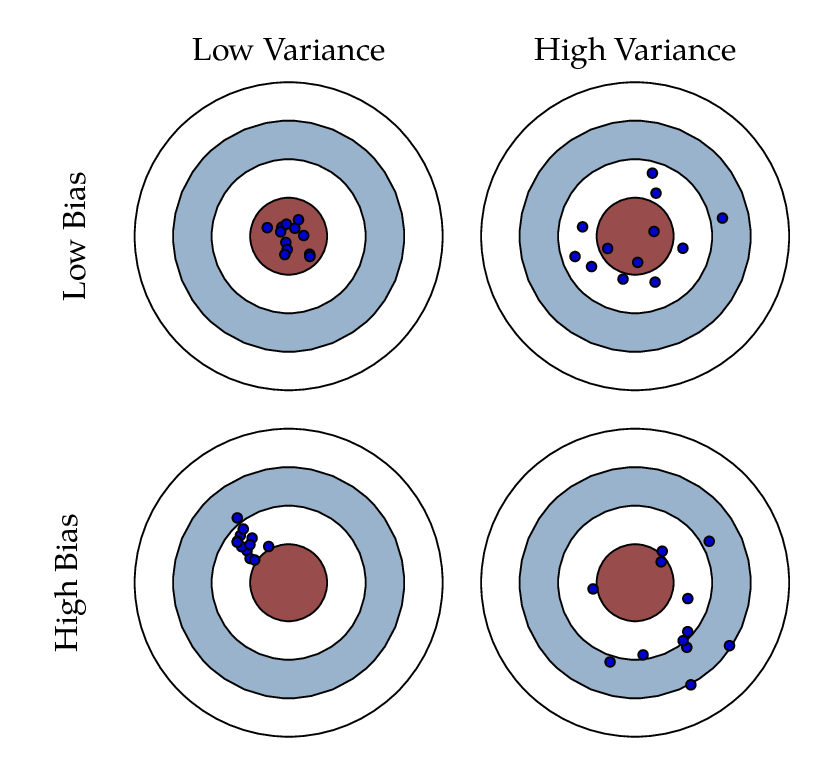## 特征工程

### 类别特征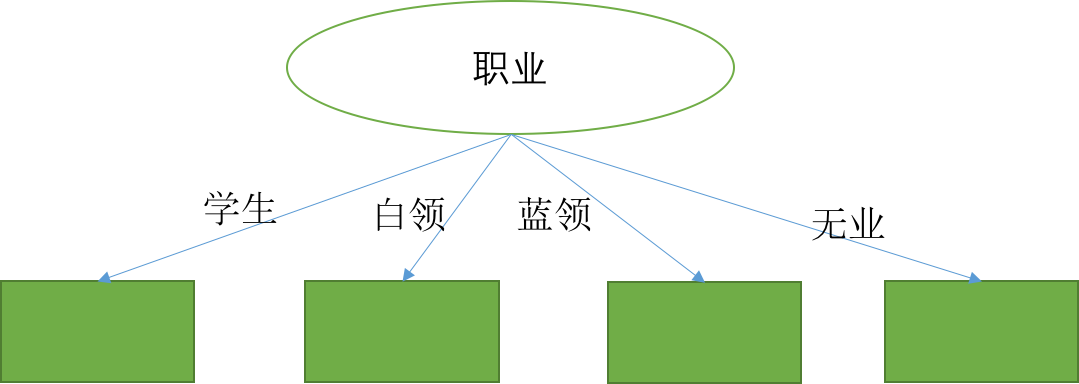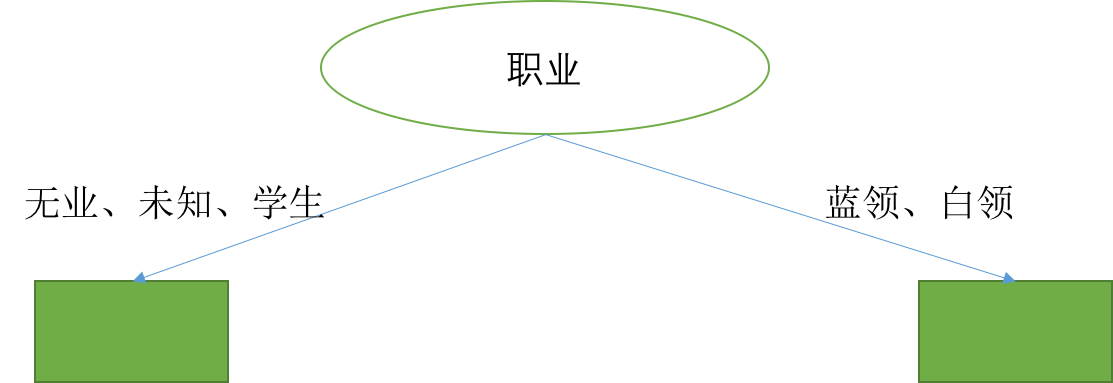## 决策树的其他分裂准则

### 信息增益率

$$IV(a) = - \sum_v \frac{|D_v|}{D} \log _ 2 \frac{|D_v|}{D}$$

$$Gain\_ ratio(D, a) = \frac{Gain(D, a)}{IV(a)}$$

### 基尼系数

\begin{align} Gini(D) &= \sum_k p_k \sum_{k' \ne k} p_{k'} \\ &=\sum_k p_k(1-p_k) \\ &=1 - \sum_k p_k^2 \end{align}

## 复现代码

### 过拟合现象代码

import numpy as np
import pandas as pd
import matplotlib.pyplot as plt

from sklearn.tree import DecisionTreeClassifier

mask = np.random.randn(iris.data.shape) < 0.8 # 20% for test

train_err = []
test_err = []
depths = range(1, 10)
for n in depths:
clf = DecisionTreeClassifier(max_depth=n)
clf.fit(train_x, train_y)
train_err.append(1 - clf.score(train_x, train_y))
test_err.append(1 - clf.score(test_x, test_y))

plt.plot(depths, train_err, '.-')
plt.plot(depths, test_err, '.-')

plt.legend(['train error', 'test error'])
plt.xlabel('depth')
plt.ylabel('error')

plt.show()


## 思考与实践

1. 举例说明在最终的决策树中，缺失值样本在所有叶子节点中的权重之和等于1！
2. 实现k折叠交叉验证，选择最佳决策树模型，最佳模型参数包括决策树深度、叶子节点上最少样本数目、分裂准则这三个。
import numpy as np
import pandas as pd
import matplotlib.pyplot as plt

from sklearn.tree import DecisionTreeClassifier
from sklearn.cross_validation import KFold

mask = np.random.randn(iris.data.shape) < 0.8 # 20% for test

"""实现K-Fold算法

"""
best_err = 1
best_params = {'depth':0, 'min_samples_leaf': 1, 'criterion': 'entropy'}
k = 5
clf = DecisionTreeClassifier()

### YOUR CODE, 定义 kfold 对象

for depth in range(1,10):
for min_samples_leaf in [1,3,10,30]:
for criterion in ['entropy', 'gini']: